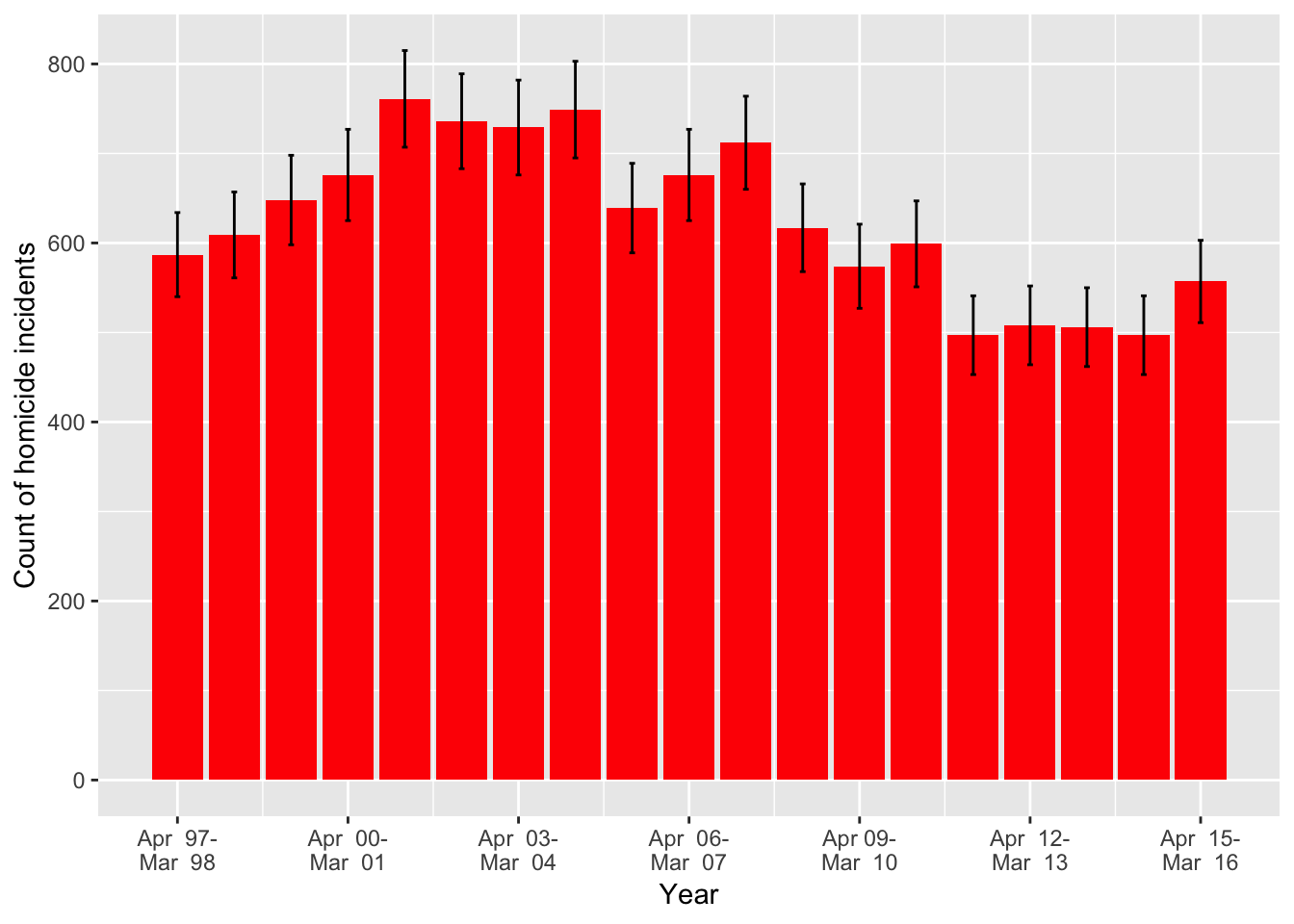Data are from Office for National Statistcs Homicide data, 2017. [Note the total for the last three years, from April 2013 to March 2016,is 1,560, whereas this total is reported as 1,545 in the preceding analysis for Figure 8.5 on page 225. This accords with the data provided by ONS]

library(ggplot2)
p <- ggplot(df, aes(x=Year, y=CountOfHomicideIncidents)) # initial plot
p <- p + geom_bar(stat="identity", fill="red") # assign bar chart type
yearLabels <- c("Apr  97-\nMar  98","Apr  00-\nMar  01","Apr  03-\nMar  04","Apr  06-\nMar  07","Apr 09-\nMar  10","Apr  12-\nMar  13","Apr  15-\nMar  16") # assign labels for x-axis

p <- p + geom_errorbar(aes(ymin=df$Lower95CI, ymax=df$Upper95CI), width=.1) # 95% intervals

p <- p + scale_x_continuous(breaks=seq(1997, 2015, 3), labels =yearLabels) # attach labels and their break points
p <- p + scale_y_continuous(breaks=seq(0, 800, 200)) # define break points for y-axis
p <- p + labs(y="Count of homicide incidents") # add y-axis label and caption
pFigure 9.4 Number of homicides each year in England and Wales between 1998 and 2016, and 95% confidence intervals for the underlying ‘true’ homicide rate.